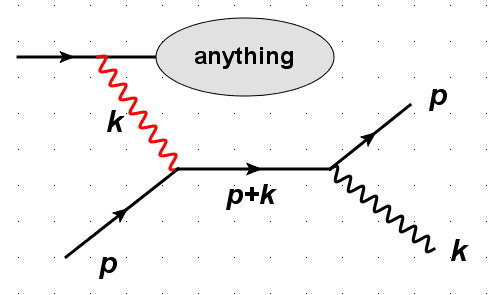# What happens if photon propagator goes on shell?

• A
• Chenkb
In summary, the conversation discusses the forward Compton scattering process and the issue of the red photon becoming a propagator with zero momentum, leading to a divergence in the cross section. It is suggested to regulate this divergence by considering a cutoff for detecting soft photons. This is discussed in QFT textbooks such as Peskin-Schröder in chapter 6. The red photon's lack of definite momentum is also compared to understanding soft or collinear divergences.

#### Chenkb

I am thinking about a problem. Consider the forward Compton scattering process e(p)+γ(k) -> e(p)+γ(k), as shown in the following figure.
If we consider the initial red photon is emitted by another electron which then goes to anything, then how can we write down the whole amplitude for this process?
The problem troubles me is that the red photon becomes a propagator with k^2 = p^2 = 0, which is divergent if in the denominator!#### Attachments

The photon will not be on-shell for anything where you can measure a momentum difference between the particles it attach to at the top.

For an actual cross section computation you have to put a cutoff on how soft photons you can detect and the divergence in the cross section due to the exchange of zero-momentum photons will be regulated by the emission of soft photons. This occurs at the cross section level, not at the amplitude level.

Formally, if you consider a series of detectors that each can measure lower and lower energy soft photons, the cross section diverges. This should be discussed in any QFT textbook, e.g., the discussion in Peskin-Schröder is in chapter 6.

Orodruin said:
The photon will not be on-shell for anything where you can measure a momentum difference between the particles it attach to at the top.

For an actual cross section computation you have to put a cutoff on how soft photons you can detect and the divergence in the cross section due to the exchange of zero-momentum photons will be regulated by the emission of soft photons. This occurs at the cross section level, not at the amplitude level.

Formally, if you consider a series of detectors that each can measure lower and lower energy soft photons, the cross section diverges. This should be discussed in any QFT textbook, e.g., the discussion in Peskin-Schröder is in chapter 6.
Thanks a lot. This reminds me that the red photon no-longer has a definite momentum, and the situation is similar to the case of understanding soft or collinear divergences.